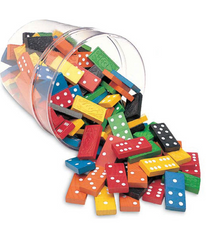Emergent Learner

• Add both sides of a domino to find total. Draw total number of dots and write numeral.
• Make a domino train by counting dots using 1 to 1 correspondence.
• Count dots on one side of the tile and match with another tile with same pattern. Find the numeral card to represent the number of dots.
• Double 6 dominoes in pairs, students choose a domino tile and counts forwards and backwards using the dots on the tile.
• Students need to pick 2 dominoes and line the matching dots next to each other. Aim is to get the longest line.

Perceptual Learner

• Take 2 domino tiles. Cover one and find total.
• Take one domino tile. Friend covers one side and student must visualise to add.
• Double 6 domino box. Flash partner a domino tile. How many did you see? If they are correct they keep the tile. Continue taking turns. Person with the most tiles at the end is the winner.
• Children pick 2 numeral cards (1-6). They must think about how it might look on a domino tile and then make a domino tile using those numbers.

Figurative Learner

• Take 2 domino tiles, 1 dot domino and 1 numeral domino. Start at numeral domino, add and count on using dots on 2nd tile.
• Have a pile of dominoes facing down. Take turns to turn a tile over. If you get a double, record number sentence and keep tile. If it is not a double put it in a separate pile. Person with the most doubles is the winner.
• Students turn a numeral card and a 6 dotted domino. Add and explain how.
• Place dominoes face down. Turn one and add together.  Record number. Partner holds up 1 to 5 fingers on their hand. Child must use their recorded number to count on the number displayed on the hand.

Counting On

• Turn 2 numeral dominoes and add them together.
• Have a pile of dominoes facing down. take turns to turn a tile over. If you get a double or near double, record number sentence and keep tile.  If it is not a double or near double put it in a separate pile. Person with the most tiles is the winner.
• Double 6 domino box. Turn 2 dominoes and race to see who can add the quickest. Winner must verbalise strategy to friend.
• Double 9 numeral dominoes. In pairs, students choose a numeral domino and read it as a 2 digit number. Take away 10 and record on an empty number line.
• Flip two dominoes and add them quickly.

Facile

• Turn 1 numeral domino. First number on the tile to represent 10. If a domino has a 7 on one side and a 6 on the other it becomes 76. Students work out how many more to 100.
• Students are given a target number and use dominoes to add or subtract to get target number.
• Flip 3 or more dominoes and add them together.
• In pairs, students choose 2 numeral dominoes and read them as 2 digit numbers. Use split strategy to add tens and ones separately.

• Naomi Countryman